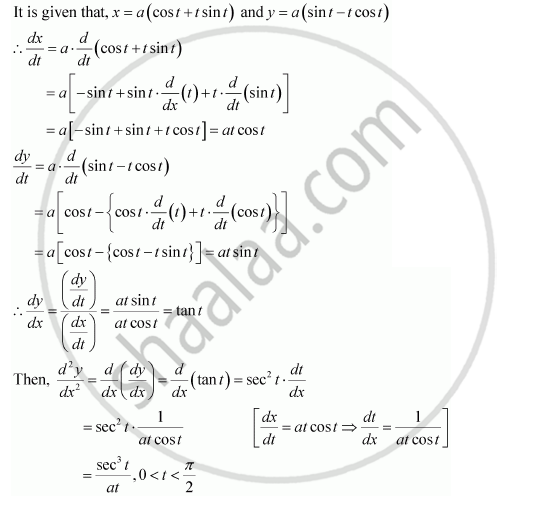Share

# If X = a (Cos T + T Sin T) and Y = a (Sin T – T Cos T), Find (D^2y)/Dx^2 - CBSE (Commerce) Class 12 - Mathematics

#### Question

If x = a (cos t + t sin t) and y = a (sin t – t cos t), find (d^2y)/dx^2

#### SolutionIs there an error in this question or solution?

#### APPEARS IN

NCERT Solution for Mathematics Textbook for Class 12 (2018 to Current)
Chapter 5: Continuity and Differentiability
Q: 17 | Page no. 192

#### Video TutorialsVIEW ALL 

Solution If X = a (Cos T + T Sin T) and Y = a (Sin T – T Cos T), Find (D^2y)/Dx^2 Concept: Logarithmic Differentiation.
S